# Consider the following figure of line MN. Say whether following statements are true or false in context of the given figure.

(a) Q, M, O, N, P are points on the line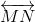.

(b) M, O, N are points on a line segment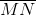.

(c) M and N are end points of line segment.

(d) O and N are end points of line segment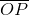.

(e) M is one of the end points of line segment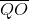.

(f) M is point on ray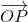.

(g) Rayis different from ray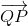.

(h) Rayis same as ray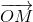.

(i) Rayis not opposite to ray.

(j) O is not an initial point of(k) N is the initial point of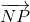and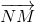.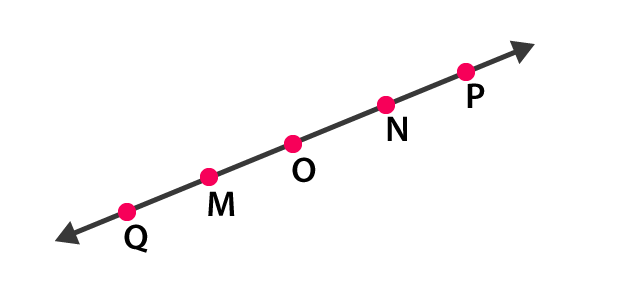Solution

(a)True

Q, M, O, N, P are points on the line MN.

(b) True

M, O, N are points on a line segment MN .

(c) True

M and N are end points of line segment MN .

(d) False

O and N are end points of line segment OP .

(e) False

M is one of the end points of line segment QO .

(f) False

M is point on ray OP.

(g) True

Ray OP is different from ray QP

(h) False

Ray OP is same as ray OM.

(i) False

Ray OM is not opposite to ray OP

(j) False

O is not an initial point of OP

(k) True

N is the initial point of NP and NM.(0)(0)